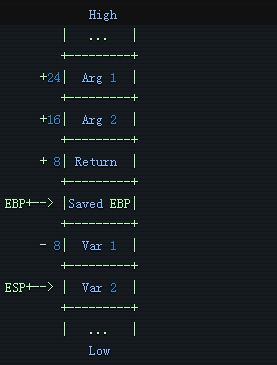# 开发一个Linux调试器（八）：堆栈展开

void a() {
//stopped here
}
void b() {
a();
}
void c() {
a();
}
int main() {
b();
c();
}

* frame #0: 0x00000000004004da a.out`a() + 4 at bt.cpp:3
frame #1: 0x00000000004004e6 a.out`b() + 9 at bt.cpp:6
frame #2: 0x00000000004004fe a.out`main + 9 at bt.cpp:14
frame #3: 0x00007ffff7a2e830 libc.so.6`__libc_start_main + 240 at libc-start.c:291
frame #4: 0x0000000000400409 a.out`_start + 41void debugger::print_backtrace() {

auto output_frame = [frame_number = 0] (auto&& func) mutable {
std::cout << "frame #" << frame_number++ << ": 0x" << dwarf::at_low_pc(func)
<< ' ' << dwarf::at_name(func) << std::endl;
};

auto current_func = get_function_from_pc(get_pc());
output_frame(current_func);

auto frame_pointer = get_register_value(m_pid, reg::rbp);

while (dwarf::at_name(current_func) != "main") {
output_frame(current_func);
}
}

void debugger::print_backtrace() {
auto output_frame = [frame_number = 0] (auto&& func) mutable {
std::cout << "frame #" << frame_number++ << ": 0x" << dwarf::at_low_pc(func)
<< ' ' << dwarf::at_name(func) << std::endl;
};
auto current_func = get_function_from_pc(get_pc());
output_frame(current_func);
auto frame_pointer = get_register_value(m_pid, reg::rbp);
while (dwarf::at_name(current_func) != "main") {
output_frame(current_func);
}
}

else if(is_prefix(command, "backtrace")) {
print_backtrace();
}